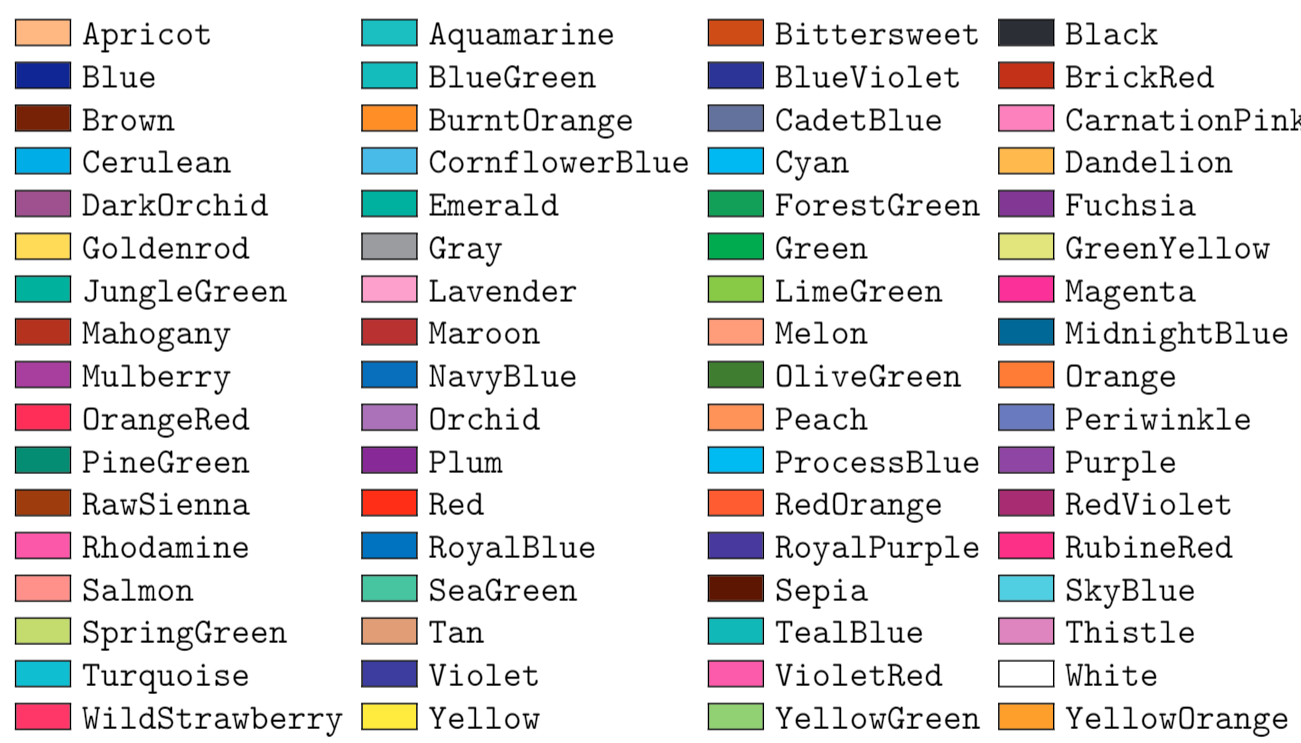# LaTeX font size command in Caption

jospercomp shared this question 2 years ago

Will it be possible to use the Latex font in Caption to individually change the size of the letters in Caption?

\tiny \large\scriptsize \Large\footnotesize \LARGE\small \huge\normalsize \HugeYou can use mathmagic's syntax, (basically use $at the beginning and the end of the LaTeX string. You can also use \text{} instead of \mbox{}) or if the caption is for an input box, create a text and use it as caption of the input box. (Right click on the input box in the attached file to see its basic properties, and the text that is currently its caption). ## Comments (9)2 try$\mbox{\tiny esto es lo} \mbox{\large que quieres?}$in a caption1$ \mbox{\Large %v} \mbox{\LARGE \textbf{%v} \mbox{\Large %f} \mbox{\Large %d} \mbox{\Large %n}$https://wiki.geogebra.org/e...1$ \mbox{\Large \textbf{A}}$1 SetCaption(A, "$ \mbox{\LARGE \textbf{"+Element(l1,1)+"}}$" ) SetCaption(B, "$ \mbox{\LARGE \textbf{"+Element(l1,2)+"}}$" ) SetCaption(C, "$ \mbox{\LARGE \textbf{"+Element(l1,3)+"}}$" ) SetCaption(A', "$ \mbox{\LARGE \textbf{"+Element(l1,1)+"}}$" ) SetCaption(B', "$ \mbox{\LARGE \textbf{"+Element(l1,2)+"}}$" ) SetCaption(C', "$ \mbox{\LARGE \textbf{"+Element(l1,3)+"}}$" ) SetCaption(A'_{1}, "$ \mbox{\LARGE \textbf{"+Element(l1,1)+"}}$" ) SetCaption(B'_{1}, "$ \mbox{\LARGE \textbf{"+Element(l1,2)+"}}$" ) SetCaption(C'_{1}, "$ \mbox{\LARGE \textbf{"+Element(l1,3)+"}}$" )1 LaTeX font size \tiny \scriptsize \footnotesize \small \normalsize \large \Large \LARGE \huge \Huge1$ \mbox{\Large \textbf{%n=%v}}$1$ \mbox{\color{Red} \large \textbf{%n}} \mbox{\color{Red} \Large \textbf{%n}} \mbox{\color{Red} \LARGE \textbf{%n}} \mbox{\color{Red} \huge \textbf{%n}} \mbox{\color{Red} \Huge \textbf{%n}}$LaTeX Color2 You can use mathmagic's syntax, (basically use$ at the beginning and the end of the LaTeX string. You can also use \text{} instead of \mbox{}) or if the caption is for an input box, create a text and use it as caption of the input box.

(Right click on the input box in the attached file to see its basic properties, and the text that is currently its caption).1

Thank you so much!!!